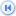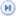Spike's Calculators

# Length Units

This is a list of the units used in length conversions. It includes Metric, Imperial, and US customary systems length units with a short description were available and their Imperial and Metric values.

Unit NameConversion typeSystemNoteImperial/USMetric
angstrom (∆)LengthMetricunit of measurement of the wavelength of light1 angstrom (∆) = 3.28e-10 feet (ft)1 angstrom (∆) = 1.0e-10 metres (m)
arpent (Quebec)LengthCanadian, FrenchOne of five old french units that are allowed to be used in Canada1 arpent (Quebec) = 191.835feet (ft)1 arpent (Quebec) = 58.47 metres (m)
astronomical unit (au)LengthAstronomicalOne astronomical unit (au) is equal to the average distance between the Earth and the Sun1 astronomical unit (au) = 4.908e+11 feet (ft)1 astronomical unit (au) = 1.496e+11 metres (m)
atomic unit of length (a0)LengthAtomica unit in the electron's world. One atomic unit of length, a physical constant, equal to the radius of a Bohr1 atomic unit of length (a0) = 1.736e-10 feet (ft)1 atomic unit of length (a0) = 5.292e-11 metres (m)
attometre (am)LengthMetricatto was added to the metric prefixes in 19641 attometre (am) = 3.281e-18 feet (ft)1 attometre (am) = 1.0e-18 metres (m)
cable length (cbl)LengthImperiala measure used at sea by naval authorities1 cable length (cbl) = 6.076e+02 feet (ft)1 cable length (cbl) = 1.852e+02 metres (m)
centimetre (cm)LengthMetricthe centimetre (cm) was the base unit in the CGS system of units1 centimetre (cm) = 0.0328 feet (ft)1 centimetre (cm) = 0.01 metres (m)
chain (ch)LengthImperiala length unit used by surveyors1 chain (ch) = 66 feet (ft)1 chain (ch) = 20.1168 metres (m)
chain (ch) U.S. surveyLengthUS Customaryunit used by surveyors based on the US survey foot1 chain (ch) U.S. Survey = 66.000132 feet (ft)1 chain (ch) U.S. Survey = 20.11684 metres (m)
decametre (dam)LengthMetricrarely used metric length measurement.1 decametre (dam) = 32.8 feet(ft)1 decametre (dam) = 10 metres (m)
decimetre (dm)LengthMetricrarely used metric length measurement.1 decimetre (dm) = 0.328 feet (ft)1 decimetre = 0.1 metres (m)
exametre (Em)LengthMetricexa was added to the metric prefixes in 19751 exametre (Em) = 3.281e+18 feet (ft)1 exametre (Em) = 1.0e+18 metres (m)
femtometre (fm)LengthMetricfemto was added to the metric prefixes in 19641 femtometre (fm) = 3.281e-15 feet (ft)1 femtometre (fm) = 1.0e-15 metres (m)
foot (ft)LengthImperialone Imperial foot (ft) equals 0.999998 foot (ft) U.S. survey1 foot (ft) = 12 inches (in)1 foot (ft) = 0.3048 metres (m)
foot (ft) U.S. surveyLengthUS CustomaryMeasurement used in land surveying in the US1 foot (ft) U.S. Survey = 1.000002 feet (ft)1 foot (ft) U.S. Survey = 0.3048006096 metres (m)
french foot (Quebec)LengthCanadian, Frenchfrench foot = pied = 12.789 inches. One of five permitted old French units in Canada1 french foot (Quebec) = 1.06575feet (ft)1 french foot (Quebec) = 0.3248406metres (m)
french perch (Quebec)LengthCanadian, FrenchPerche, a Quebec land measure equal to 18 french feet 1 french perch (Quebec) = 19.1835 feet (ft)1 french perch (Quebec) = 5.847 metres (m)
furlong (fur)LengthImperialIs used to describe the various distances at a horse race. the Imperial furlong (fur) = 0.999998 furlongs (fur) U.S. survey1 furlong (fur) = 660 feet (ft)1 furlong (fur) = 201.168 metres (m)
furlong (fur) U.S. surveyLengthUS Customarybased on US survey foot1 furlong (fur) U.S. Survey = 660.00132 feet (ft)1 furlong (fur) U.S. Survey = 201.1684 metres (m)
gigametre (Gm)LengthMetricGiga is derived from the greek word "gigas" giant1 gigametre (Gm) = 3.28e+09 feet (ft)1 gigametre (Gm) = 1.0e+09 metres (m)
hand (hh)LengthImperialused in measuring the height of a horse1 hand (hh) = 0.33333 feet (ft)1 hand (hh) = 0.1016 metres (m)
hectometre (hm)LengthMetricone hectometre is the side length of a square hectare, equal to 100 metres1 hectometre (hm) = 328.084 feet (ft)1 hectometre (hm) = 100 metres (m)
inch (in)LengthImperialtraditionally the width of a thumbnail1 inch (in) = 0.08332 feet (ft)1 inch (in) = 0.0254 metres (m)
kilometre (km)LengthMetricused for measuring larger distances, odometer1 kilometre (km) = 3280.84 feet (ft)1 kilometre (km) = 1000 metres (m)
league (lea) U.S. surveyLengthUS Customaryland league, a rarely used measurement based on the US survey foot1 league (lea) U.S. Survey = 15840.032 feet1 league (lea) U.S. Survey = 4828.04 metres (m)
league (lea) landLengthImperialthe land league equals 3 miles (mi)1 league (lea) land = 15840 feet (ft)1 league (lea) land = 4828.032 metres (m)
link (li)LengthImperiala length measurement used in land surveying1 link (li) = 0.66 feet (ft)1 link (li) = 0.201168 metres (m)
megametre (Mm)LengthMetricMega means large derived from the Greek word "megas"1 megametre (Mm) = 3.2808e+06 feet (ft)1 megametre (Mm) = 1.0e+06metres (m)
metre (m)LengthMetricmetre (m) is a base unit in the Metric system1 metre (m) = 3.28084 feet (ft)1 metre (m) = 1000 millimetres (mm)
micrometre (µm)LengthMetricThe micrometre was previously known as micron1 micrometre (µm) = 3.2808e-06 feet (ft)1 micrometre (µm) = 0.000001metres (m)
mile (mi)LengthImperialused as a distance measurement and as a speed unit, miles per hour1 mile (mi) = 5280 feet (ft)1 mile (mi) = 1609.344 metres (m)
mile (mi) U.S. surveyLengthUS Customarybased on US survey foot. one International mile = 0.999998 mile (mi) U.S. Survey1 mile (mi) U.S. Survey = 5280.01056 feet (ft)1 mile (mi) U.S. Survey = 1609.34721869 metres (m)
millimetre (mm)LengthMetricunit of length equal to one thousand of a metre1 millimetre = 3.2808e-03 feet (ft)1 millimetre = 0.001 metres (m)
nanometre (nm)LengthMetricUsed in nanotechnology, the study and application of extremely small things1 nanometre (nm) = 3.2808e-09 feet (ft)1 nanometre (nm) = 1.0e-09 metres (m)
natural unit of lengthLengthNaturala physical unit of measurement based only on a universal physical constant.1 natural unit of length = 1.267e-12 feet (ft) 1 natural unit of length = 3.862e-13 metres (m)
nautical mile (nmi)LengthImperialwas defined as one minute of latitude along any line of longitude, now one nautical mile (nmi) = 1852 metres (m)1 nautical mile (nmi) = 6076.12 feet (ft)1 nautical mile (nmi) = 1852 metres
petametre (Pm)LengthMetricpeta was added to the metric prefixes in 19751 petametre (Pm) = 3.28e+15feet (ft)1 petametre (Pm) = 1.0e+15 metres (m)
picometre (pm)LengthMetrica picometre used to be called a micromicron1 picometre (pm) = 3.2808e-12 feet (ft)1 picometre (pm) = 1.0e-12 metres (m)
planck length (LP)LengthPlanck smallest measurable length1 planck length (LP) = 5.303e-35 feet (ft)1 planck length (LP) = 1.616255e-35 metres (m)
rod (rd)LengthImperiallength unit that is the same as a pole1 rod (rd) = 16.5 feet (ft)1 rod (rd) = 5.0292 metres (m)
rod (rd) U.S. surveyLengthUS Customarymeasure based on the US survey foot.rod = perch1 rod (rd) U.S. Survey = 16.500033 feet (ft)1 rod (rd) U.S. Survey = 5.02921005842 metres (m)
thou (th)LengthImperialequal to one thousandth of an inch. Mil in the US1 thou (th) = 8.3332E-05 feet (ft)1 thou (th) = 0.0000254 metres (m)
yard (yd)LengthImperialthe Imperial metre1 yard (yd) = 3 feet (ft)1 yard (yd) = 0.9144 metres (m)
yoctometre (ym)LengthMetrica yoctometre is about as long as the diameter of a Neutrino, a neutral subatomic particle1 yoctometre (ym) = 3.2808e-24 feet (ft)1 yoctometre (ym) = 1.0e-24 metres (m)
yottametre (Ym)LengthMetricYotta is the largest decimal unit prefix in the metric system1 yottametre (Ym) = 3.28e+24 feet (ft)1 yottametre (Ym) = 1.0e+24 metres (m)
zeptometre (zm)LengthMetriczepto was added to the metric prefixes in 19911 zeptometre (zm) = 3.2812e-21 feet (ft)1 zeptometre (zm) = 1.0e-21 metres (m)
zettametre (Zm)LengthMetriczetta was added to the metric prefixes in 19911 zettametre (Zm) = 3.28e+21 feet (ft) 1 zettametre (Zm) = 1.0e+21 metres (m)